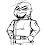## Friday, November 23, 2018

### Excel Has More Functions Than Any One Person Likely Ever Learn

Like all the Smalltalk classes.  Not sure if the words in this post will help other Excel users find this, but it's worth a try.

I have this spreadsheet where I subtotal each decade's mass murders.  One column contains the count of the decade's rows, in this example, rows 2-5.  The count is in in B6.  The row count is computed with =ROWS(B2:B5).  I would like to do an operation on just the cells in that decade's rows, but not including the subtotal row's cell.  The SUMIF and SUMIFS functions are very useful, if you know the range of rows and the column.  If you are doing an operation in your subtotal row, the current row number =ROW().  Put the decade's starting row number in AH6 with the formula =ROW()-B6.  Put the column  number for AD (from which I want to construct a range for the SUMIF function, in AJ6.  To get the contents of that cell, =INDIRECT(ADDRESS(AH6,AJ6)).  ADDRESS converts row and column number to a cell address and INDIRECT returns the contents of that cell.  Best of all, I can copy this formula (or a calculated range) into every subtotal row, without making it specific to that row.

#### 1 comment:

1.I could have used some of that. I was making a Sudoku Solver in the Open Office spreadsheet and I basically had to hand-code all of the equation addresses because I couldn't auto-fill them (Scaling issues of changing from a 9x9 grid to a 9x9x9 grid for ticking off possible answers.)